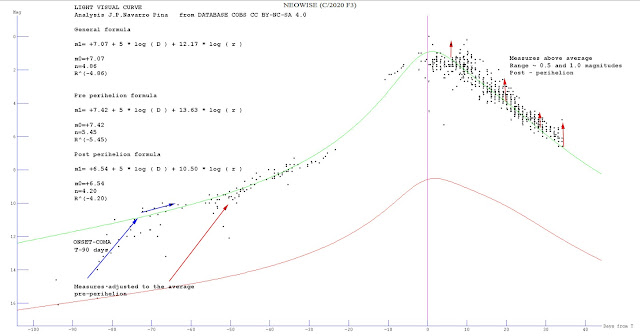## lunes, 23 de septiembre de 2019

### Tuning the diameter of the comet's nucleus C/2018 W2 AFRICANO

I find a new correlation of diameter and magnitude absolute , there are several applicable equations to calculate based on the absolute luminosity of a comet, its diameter or its nuclear radius : Delsemme 1987 r nuc = 10^( 1.59-0.199H ) applied this formula ,my calculations indicated r nuc ( C/2048W2 ) = 0.99 kms For Sosa and Fdez ( 2011 ) log D ( kms ) = 1.2-0.13H for this formula D ( kms ) = 0.79 kms assuming p=0.4 g cm3 it is necessary to compile absolute total magnitudes absolutes for all's comets .

## miércoles, 4 de septiembre de 2019

### ANALYSIS LIGHT CURVE CCD C/2018 W2 AFRICANO

Update of the ccd light curve of comet C / 2018 W2 AFRICAN based on 488 observations of this comet, where I have made the following approximate calculations: 1 Photometric parameters of the comet, calculation of the estimated magnitude estimated at m0 = + 12.5 and the rate of increase in brightness or activity index, n = 4.9, the result indicates an index that shows little or no activity at the core level, behaving as a solid object with almost no gaseous activity. 2 Based on the formula of Sosa and Fdez 2011,: log D (km) = 1.5-0.13H The estimated diameter is 0.9 kms, which is consistent with the data offered for other comets in the same study. 3 Water production rate, based on the estimated absolute magnitude, Q (H2O) ~ 27.6 mol / s, a fairly low figure that matches the tables shown in the same study. 4 Fraction of active area, here we can only establish a scale that turns out to be between 0.5### Update Light Visual Curve C/2020 F3 NEOWISE

New update of the visual light curve of comet NEOWISE, based on 798 observations of the comet, from the COBS database, my analysis includes ...